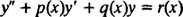## Second-Order Linear Equations

The order of a differential equation is the order of the highest derivative appearing in the equation. Thus, a second‐order differential equation is one that involves the second derivative of the unknown function but no higher derivatives.

A second‐order linear differential equation is one that can be written in the formwhere a( x) is not identically zero. [For if a( x) were identically zero, then the equation really wouldn't contain a second‐derivative term, so it wouldn't be a second‐order equation.] If a( x) ≠ 0, then both sides of the equation can be divided through by a( x) and the resulting equation written in the formIt is a fact that as long as the functions p, q, and r are continuous on some interval, then the equation will indeed have a solution (on that interval), which will in general contain two arbitrary constants (as you should expect for the general solution of a second‐order differential equation). What will this solution look like? There is non explicit formula that will give the solution in all cases, only various methods that work depending on the properties of the coefficient functions p, q, and r. But there is something definitive — and very important — that can be said about second‐order linear equations.